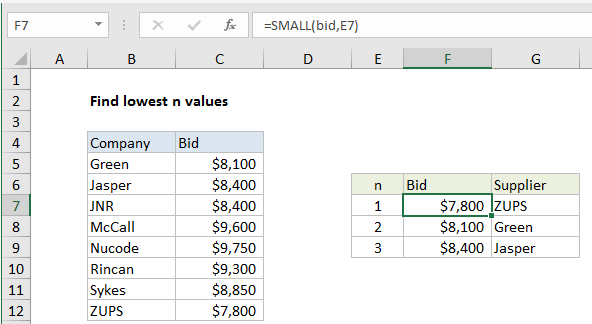## Excel Office

Excel How Tos, Tutorials, Tips & Tricks, Shortcuts

# Find lowest n values in Excel

This tutorial shows how to Find lowest n values in Excel using the example below;

## Formula

`=SMALL(range,n)`## Explanation

To find the n lowest values in a set of data, you can use the SMALL function. This can be combined with INDEX as shown below to retrieve associated values. In the example shown, the formula in F7 is:

`=SMALL(bid,E7)`

Note: this worksheet has two named ranges: bid (C5:C12), and company (B5:B12), used for convenience and readability.

Also See:   SMALL function: Description, Usage, Syntax, Examples and Explanation

### How this formula works

The small function can retrieve the smallest values from data based on rank. For example:

```=SMALL(range,1) // smallest
=SMALL(range,2) // 2nd smallest
=SMALL(range,3) // 3rd smallest```

In this case, the rank simply comes from column E.

### Retrieve associated values

To retrieve the name of the company associated with smallest bids, we use INDEX and MATCH. The formula in G7 is:

`=INDEX(company,MATCH(F7,bid,0))`

Here, the value in column F is used as the lookup value inside MATCH, with the named range bid (C5:C12) for lookup_array, and match type set to zero to force exact match. MATCH then returns the location of the value to INDEX as a row number. INDEX then retrieves the corresponding value from the named range company (B5:B12).

Also See:   Get nth match with VLOOKUP in Excel

The all-in-one formula to get company name in one step would look like this:

`=INDEX(company,MATCH(SMALL(bid,E7),bid,0))`